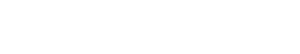# Hypothesis Testing

Hypothesis Testing is used to determine whether a null hypothesis can be rejected or not. The null hypothesis is the assumption that there is no difference between two groups. The alternative hypothesis is the claim that there is a difference between the two groups.

In order to test the null hypothesis, a statistical test is used. This test will generate a p-value, which is the probability that the null hypothesis is true. If the p-value is below a certain threshold (usually 0.05), then the null hypothesis is rejected and the alternative hypothesis is accepted.

Hypothesis Testing can be used for a variety of purposes, such as testing whether a new marketing campaign is effective, or whether a new product is better than the existing one.

Hypothesis Testing is similar to A/B Testing and Experimental Design, but there are some key differences. A/B Testing is usually used to test two different versions of a product or service, whereas Hypothesis Testing can be used to test any type of difference between two groups.

## 4 Steps of Hypothesis Testing

1. Select your null and alternative hypotheses

Null Hypothesis: There is no difference between two groups.

Alternative Hypothesis: There is a difference between the two

There are many different types of test statistics, but the most common ones are z-tests and t-tests.

A z-test is used when the population standard deviation is known. A t-test is used when the population standard deviation is unknown.

Sample calculation of p-value using t-test :

t-test = (xbar-mu)/(s/sqrt(n))

xbar = sample mean

mu = population mean

s = sample standard deviation

n = sample size

p-value = 2 * (1 – norm.cdf(abs(t-test)))

Sample calculation of p-value using z-test :

z-test = (xbar – mu)/(sigma/sqrt(n))

xbar = sample mean

mu = population mean

sigma = population standard deviation

n = sample size

p-value = 2 * (1 – norm.cdf(abs(z-test)))

If the p-value is less than 0.05, then the null hypothesis is rejected and the alternative hypothesis is accepted. This means that there is a statistically significant difference between the two groups.

If the p-value is greater than 0.05, then the null hypothesis cannot be rejected. This does not mean that the null.Unlock the power of actionable insights with AI-based natural language processing.